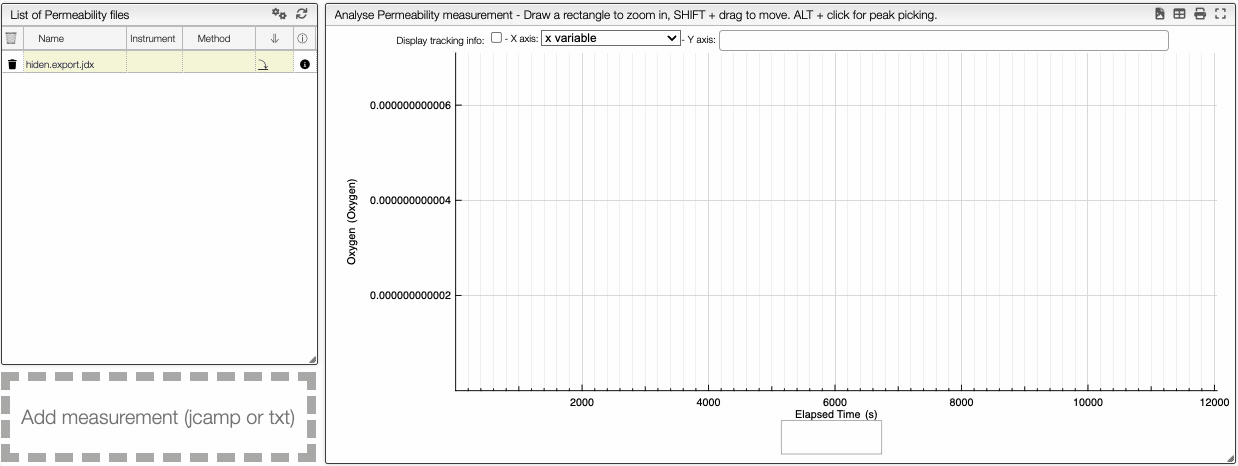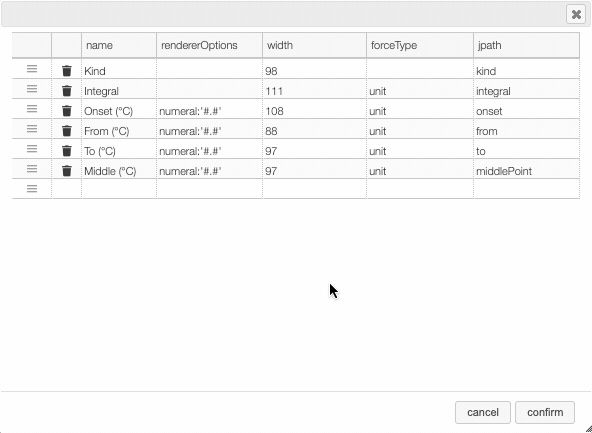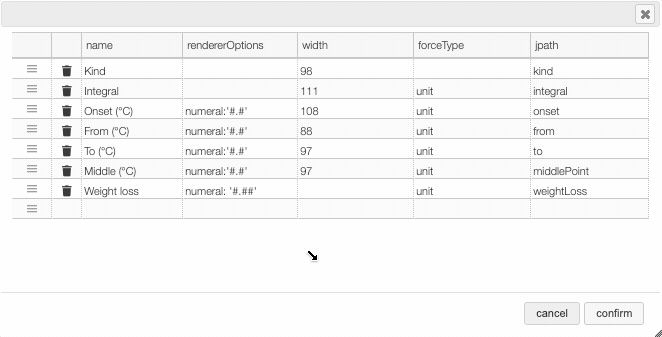# Permeability

## Theoretical background​

Permeability is a phenomenon where molecules diffuse through a solid. This phenomenon can be modeled by Fick's law of diffusion.

### Fick's law of diffusion​

The flux (or flow of mass) is proportional to the gradient of the concentration. In one dimension, Fick's first law of diffusion if given by,

$J = -D \frac{\partial \phi}{\partial x}$

Where $J$ is the flux, $D$ is the mass diffusivity, and $\phi$ is the concentration of gas. The above equation can be approximated by the relation

$J = -D \frac{(C_2 - C_1)}{\delta}$

Where $C$ is the concentration at the edge and $\delta$ is the thickness of the membrane.

You can upload a spectrum either as JCAMP or TXT format.

## Spectra analysis​

In the graph view, it is possible to superimpose multiple y variables, in order to have a more global view of the different variables.

Plot multiple y variables
You can superimpose multiple curve on the same plot.

This tool allows you to visualize multiple entries on the same graph. For example, the permeability of methane, nitrogen, and oxygen can be visualized simultaneously. You can select, in the y axis menu, the variables that you want to see.Every curve will be plotted with a different color, and you can zoom in by selecting the area you want and zoom out by double-clicking on the graph. Additionally, you can hide and show curves by clicking on the corresponding eye at the bottom of the graph.

You can also apply mathematical processing to the curves via the preprocessing panel.

Spectrum preprocessing
Apply mathematical tools to the spectra.

## Preprocessing​

You can apply the following modifications to the spectra to enhance the visualization. The modifications include the following:

• Center Mean : subtract the mean from every variable observation in the dataset, so that the new variable's mean is centered at 0.
• Divide by SD : divide every variable observable in the dataset by the standard deviation yields a distribution with a standard deviation equal to 1.
• Divide by max Y : divide every value by the maximum y-value shifts all the y-values between 0 and 1.
• Rescale (x to y) : rescale the graph such that the y-values fit between specified minimum and maximum values.
• Normalize (sum to n) : normalize the integral under the curve so that it sums to n.
• Multiply (value) : multiply every y-value by a scalar.
• Add (value) : add a scalar to every y-value.
• AirPLS baseline : baseline correction using adaptive iterative reweighed penalized least squares algorithm.
• Rolling average baseline :
• Iterative polynomial baseline : baseline correction using iterative polynomial fitting algorithm.
• Rolling ball baseline :
• Rolling median baseline :

A certain range of x-values can be selected to show only a part of the spectrum using Range.

Depending on the analysis, some regions should be removed using Exclusions in order to improve the visualization.Columns customization
In many tables it is possible to select which columns to display. This is achieved by clicking on the icon.

After clicking on the icon a dialog box opens that allows to add a new column.

There are 5 parameters to fill for a new column:

• name: the column name. This will be displayed as the header to the column.
• rendererOptions: options that allows among other to format numbers. One very useful formatter is numeral (see after)
• width: number of pixels for the specific column. May stay empty for automatic layout.
• forceType: select how to display complex values (see later)
• jpath: where to find the information to display in the column

In order to add a new column you need to select the jpath using the hierarchical drop down menu.Columns can be moved or rearranged as well.rendererOptions: numeral

number,

Value to formatrendererOptionsResult
12.345678numeral:'#.##'12.34
12.3numeral:'#.00'12.30
0.3numeral:'#.0 %'30.0 %

forceType

In the database some values are stored as an object that needs to be displayed to the user in an intuitive way.

For example the unit type will store in the database the value using as units SI (we convert the data to the units defined by the 'standard international') and specify in which units the user would like to display the data. So in the following example we are in fact storing the value -150°C by storing the value in SI (Kelvin) and specifying that the user wants to see it in °C.

{  "SI": 123.15,  "unit": "°C"}

Another example is the valueUnits type that will store the data in 2 different properties (value and unit). In this case the value is stored in the specified units and there is no conversions.

{  "value": 123,  "units": "°C"}

While this way to store the data in the database is very practical it is not the way that the user would like to see his results. We have therefore the possibility to forceType: define how the user would like to see the results.

Other types include mf. This formatter allows to correctly display a molecular formula that is stored in the database as "C10H20O3". i.e. it will put the numbers in subscript (C₁₀H₂₀O₃).

1. Permeability